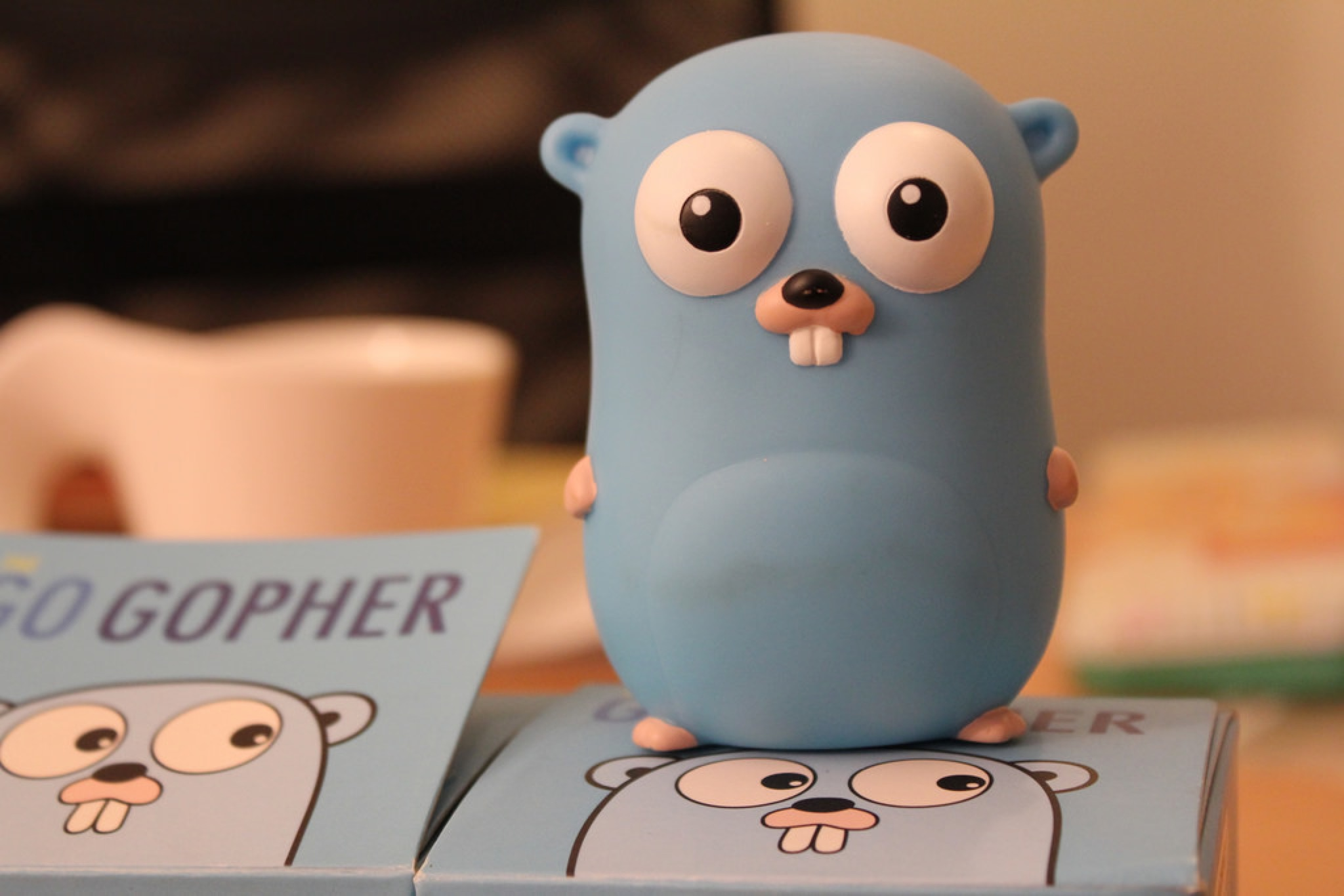``````{
"foo": "Hello, World!",
"bar": {
"b": {
"go": "1"
},
"a": {
"go": "2"
},
"c": {
"go": "3"
}
}
}``````

``````type Foobar struct {
Foo  string `json:"foo"`
Bar map[string]struct {
Go string `json:"go"`
} `json:"bar"`
}``````

1. 按顺序输出 `bar.a``bar.b``bar.c`
2. 按顺序输出 `bar.b``bar.a``bar.c`

When iterating over a map with a range loop, the iteration order is not specified and is not guaranteed to be the same from one iteration to the next. Since the release of Go 1.0, the runtime has randomized map iteration order.

## 实现类似 SortedMap 的遍历

SortedMap 主要是对 key 排序，那么我们便可将 map 的 key 全部拿出来，放到一个数组中，然后对这个数组排序后对有序数组遍历，再间接取 map 里的值就行了。

``````package main

import (
"fmt"
"sort"
)

func main() {
m := make(map[string]string)
m["b"] = "2"
m["a"] = "1"
m["c"] = "3"

keys := make([]string, 0, len(m))
for k, _ := range m {
keys = append(keys, k)
}

sort.Strings(keys)

for _, k := range keys {
fmt.Printf("Key:%+v, Value:%+v\n", k, m[k])
}
}
``````

https://play.golang.org/p/vzpwizlRYUO

``````Key:a, Value:1
Key:b, Value:2
Key:c, Value:3``````

``````func sortedMap(m map[string]interface{}, f func(k string, v interface{})) {
var keys []string
for k := range m {
keys = append(keys, k)
}
sort.Strings(keys)
for _, k := range keys {
f(k, m[k])
}
}
``````

``````func main() {
m := make(map[string]interface{})
m["b"] = "2"
m["a"] = "1"
m["c"] = "3"

sortedMap(m, func(k string, v interface{}) {
val := v.(string)
fmt.Printf("Key:%+v, Value:%+v\n", k, val)
})
}``````

If you can, you should avoid using this package.

``````package main

import (
"fmt"
"github.com/virtuald/go-ordered-json"
)

func main() {
jsonString := `{
"b": "2",
"a": "1",
"c": "3"
}`

oo := json.OrderedObject{}
err := json.Unmarshal([]byte(jsonString), &oo)
if err != nil {
panic(err)
}
fmt.Printf("%+v", oo)
}
``````

``[{Key:b Value:2} {Key:a Value:1} {Key:c Value:3}]``

（你可能会怀疑是不是因为用了 `fmt.Printf` 导致打印出来的顺序变了，你可以自己遍历试一下哦~）

``````{
"foo": "Hello, World!",
"bar": {
"b": {
"go": "1"
},
"a": {
"go": "2"
},
"c": {
"go": "3"
}
}
}``````

``[{Key:foo Value:Hello, World!} {Key:bar Value:map[a:map[go:2] b:map[go:1] c:map[go:3]]}]``

``````type Foobar struct {
Foo string             `json:"foo"`
Bar json.OrderedObject `json:"bar"`
}``````

``````package main

import (
"fmt"
"github.com/virtuald/go-ordered-json"
)

func main() {

jsonString := `{
"foo": "Hello, World!",
"bar": {
"b": {
"go": "1"
},
"a": {
"go": "2"
},
"c": {
"go": "3"
}
}
}`

foobar := Foobar{}
err := json.Unmarshal([]byte(jsonString), &foobar)
if err != nil {
panic(err)
}

for _, value := range foobar.Bar {
m := value.Value.(map[string]interface{})
for k, v := range m {
fmt.Printf("%+v.%+v=%+v\n", value.Key, k, v)
}
}

}``````

``````b.go=1
a.go=2
c.go=3``````

## encoding/json

``````package main

import (
"encoding/json"
"fmt"
)

func main() {
jsonString := `{
"foo": {
"b": "1",
"a": "2",
"c": "3"
}
}`
o := make(map[string]map[string]string)
err := json.Unmarshal([]byte(jsonString), &o)
if err != nil {
panic(err)
}

fmt.Println("range:")
for _, m := range o {
for k, v := range m {
fmt.Printf("%s, %s\n", k, v)
}
}

bytes, err := json.Marshal(o)
if err != nil {
panic(err)
}

fmt.Println("")
fmt.Println("json:")
fmt.Printf("%s\n", bytes)
}``````

https://play.golang.org/p/JcTWRlYjQ5j